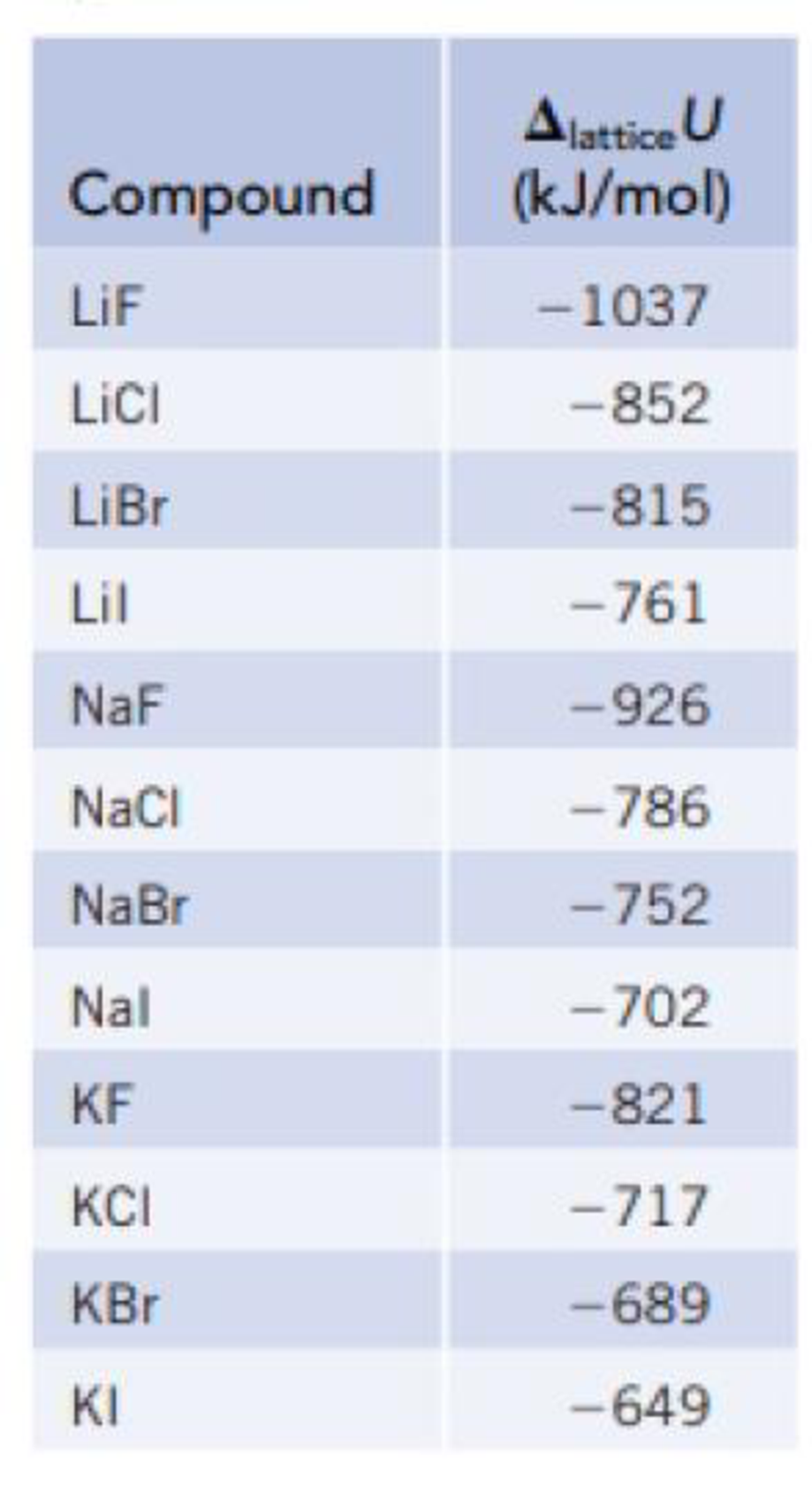Chapter 12, Problem 14PS

Chapter
Section
Textbook Problem

Examine the trends in lattice energy in Table 12.1. The value of the lattice energy becomes somewhat more negative on going from NaI to NaBr to NaCl, and all are in the range from −700 to −800 kJ/mol. Suggest a reason for the observation that the lattice energy of NaF (Δlattice U = −926 kJ/mol) is much more negative than those of the other sodium halides.TABLE 12.1Lattice Energies of Some Ionic CompoundsInterpretation Introduction

Interpretation:

The reason behind the lattice energy of sodium fluoride is more negative than the other sodium halides has to be explained.

Concept introduction:

Lattice formation enthalpy is the enthalpy change when one mole of solid crystal is formed from its scattered gaseous ions.

Explanation

The lattice energy of sodium fluoride is more negative than the other sodium halides because, lattice energy is mainly depends on the charge and size of the ions. Lattice built from smaller ions leads to more negative for the lattice energy...

Still sussing out bartleby?

Check out a sample textbook solution.

See a sample solution

The Solution to Your Study Problems

Bartleby provides explanations to thousands of textbook problems written by our experts, many with advanced degrees!

Get Started